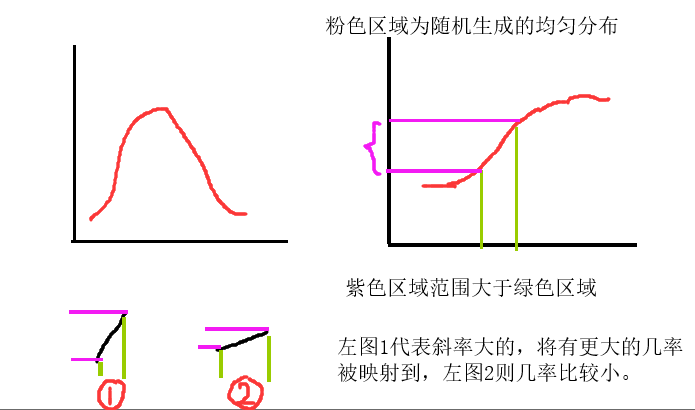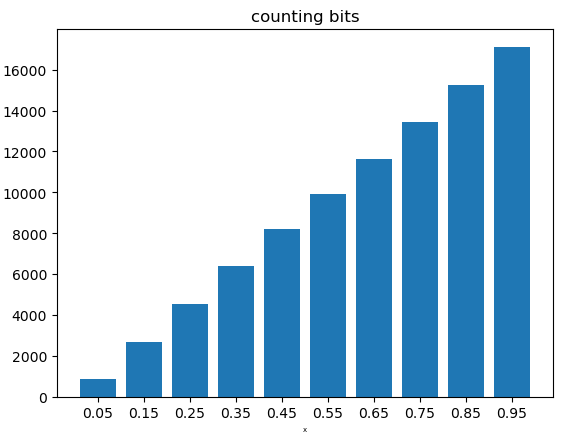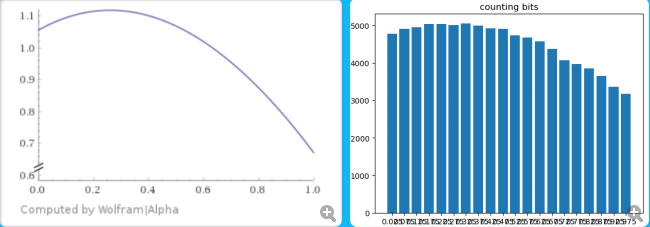• 实现的方法可以不止一种： ...本篇介绍根据累积概率分布函数的逆函数(2：invert the CDF)生成的方法。 原参考网站：https://blog.demofox.org/2017/08/05/generating-random-numbers-from-a-speci...
实现的方法可以不止一种：

rejection sampling
invert the cdf
Metropolis Algorithm (MCMC)

本篇介绍根据累积概率分布函数的逆函数(2：invert the CDF)生成的方法。
原参考网站：https://blog.demofox.org/2017/08/05/generating-random-numbers-from-a-specific-distribution-by-inverting-the-cdf/
自己的理解不一定正确，有错误望指正。
目标：
已知 y=pdf(x），现想由给定的pdf, 生成对应分布的x
PDF是概率分布函数，对其积分或者求和可以得到CDF（累积概率分布函数），PDF积分或求和的结果始终为1
步骤（具体解释后面会说）：
1、根据pdf得到cdf
2、由cdf得到inverse of the cdf
3、对于给定的均匀分布[0,1),带入inverse cdf，得到的结果即是我们需要的x
求cdf逆函数的具体方法：
对于上面的第二步，可以分成两类：
1、当CDF的逆函数好求时，直接根据公式求取，
2、反之当CDF的逆函数不好求时，用数值模拟方法
自己的理解：为什么需要根据cdf的逆去获得x？
原因一：
因为cdf是单调函数因此一定存在逆函数（cdf是s型函数，而pdf则不一定，例如正态分布，不单调，对于给定的y，可能存在两个对应的x，就不可逆）
原因二：
这仅是我自己的直观理解，根据下图所示（左上为pdf，右上为cdf）由步骤3可知，我们首先生成[0，1)的均匀随机数，此随机数作为cdf的y，去映射到cdf的x（若用cdf的逆函数表示则是由x映射到y），可以参考上图的右上，既然cdf的y是均匀随机的，那么对于cdf中同样范围的x，斜率大的部分将会有更大的机会被映射，因为对应的y范围更大（而y是随即均匀分布的），那么，cdf的斜率也就等同于pdf的值，这正好符合若x的pdf较大，那么有更大的概率出现（即重复很多次后，该x会出现的次数最多）
代码实现——方法一，公式法
import numpy as np
import math
import random
import matplotlib.pyplot as plt
import collections

count_dict = dict()
bin_count = 20

def inverseCDF():
"""
return the x value in PDF
"""
uniform_random = random.random()
return inverse_cdf(uniform_random)

def pdf(x):
return 2 * x

# cdf = x^2, 其逆函数很好求，因此直接用公式法
def inverse_cdf(x):
return math.sqrt(x)

def draw_pdf(D):
global bin_count
D = collections.OrderedDict(sorted(D.items()))
plt.bar(range(len(D)), list(D.values()), align='center')
# 因为映射bin的时候采用的floor操作，因此加上0.5
value_list = [(key + 0.5) / bin_count for key in D.keys()]
plt.xticks(range(len(D)), value_list)
plt.xlabel('x', fontsize=5)
plt.ylabel('counts', fontsize=5)
plt.title('counting bits')
plt.show()

for i in range(90000):
x = inverseCDF()
# 用bin去映射，否则不好操作
bin = math.floor(x * bin_count)  # type(bin): int
count_dict[bin] = count_dict.get(bin, 0) + 1

draw_pdf(count_dict)

结果：代码实现——方法二，数值法
数值模拟cdf的关键是创建lookup table，
table的size越大则结果越真实（即区间划分的个数）
import numpy as np
import math
import random
import matplotlib.pyplot as plt
import collections

lookup_table_size = 40
CDFlookup_table = np.zeros((lookup_table_size))

count_dict = dict()
bin_count = 20

def inverse_cdf_numerically(y):
global lookup_table_size
global CDFlookup_table
value = 0.0
for i in range(lookup_table_size):
x = i * 1.0 / (lookup_table_size - 1)
value += pdf2(x)
CDFlookup_table[i] = value
CDFlookup_table /= value  # normalize the cdf

if y < CDFlookup_table:
t = y / CDFlookup_table
return t / lookup_table_size
index = -1
for j in range(lookup_table_size):
if CDFlookup_table[j] >= y:
index = j
break
# linear interpolation
t = (y - CDFlookup_table[index - 1]) / \
(CDFlookup_table[index] - CDFlookup_table[index - 1])
fractional_index = index + t  # 因为index从0开始,所以不是 (index-1)+t
return fractional_index / lookup_table_size

def inverseCDF():
"""
return the x value in PDF
"""
uniform_random = random.random()
return inverse_cdf_numerically(uniform_random)

def pdf2(x):
return (x * x * x - 10.0 * x * x + 5.0 * x + 11.0) / (10.417)

def draw_pdf(D):
global bin_count
D = collections.OrderedDict(sorted(D.items()))
plt.bar(range(len(D)), list(D.values()), align='center')
value_list = [(key + 0.5) / bin_count for key in D.keys()]
plt.xticks(range(len(D)), value_list)
plt.xlabel('x', fontsize=5)
plt.ylabel('counts', fontsize=5)
plt.title('counting bits')
plt.show()

for i in range(90000):
x = inverseCDF()
bin = math.floor(x * bin_count)  # type(bin): int
count_dict[bin] = count_dict.get(bin, 0) + 1

draw_pdf(count_dict)

真实函数与模拟结果扩展：生成伯努利、正太分布
import numpy as np
import matplotlib.pyplot as plt
"""
reference:
https://blog.demofox.org/2017/07/25/counting-bits-the-normal-distribution/
"""

def plot_bar_x():
# this is for plotting purpose
index = np.arange(counting.shape)
plt.bar(index, counting)
plt.xlabel('x', fontsize=5)
plt.ylabel('counts', fontsize=5)
plt.title('counting bits')
plt.show()

# if dice_side=2, is binomial distribution
# if dice_side>2 , is multinomial distribution
dice_side = 2
# if N becomes larger, then multinomial distribution will more like normal distribution
N = 100

counting = np.zeros(((dice_side - 1) * N + 1))

for i in range(30000):
sum = 0
for j in range(N):
dice_result = np.random.randint(0, dice_side)
sum += dice_result

counting[sum] += 1

# normalization
counting /= np.sum(counting)
plot_bar_x()




展开全文概率密度
• 因此，这意味着还必须有某种算法来生成随机数。如果有一个程序可以生成随机数，则可以预测它，因此它并不是真正的随机数。通过生成算法生成的随机数称为伪随机数。我们可以做真正的随机数吗？为了在我们的计算机上...
1、什么是随机数(Random Numbers)?随机数并不意味着每次都有不同的数字。 随机意味着无法在逻辑上预测的事物。2、伪随机和真随机计算机在程序上工作，程序是权威的指令集。 因此，这意味着还必须有某种算法来生成随机数。如果有一个程序可以生成随机数，则可以预测它，因此它并不是真正的随机数。通过生成算法生成的随机数称为伪随机数。我们可以做真正的随机数吗？为了在我们的计算机上生成一个真正的随机数，我们需要从某个外部来源获取随机数据。 外部来源通常是我们的击键，鼠标移动，网络数据等。我们不需要真正的随机数，除非它与安全有关(如，加密密钥)或应用的基础是随机性(如，数字轮盘赌轮)。在本教程中，我们将使用伪随机数。3、生成随机数NumPy提供了random模块来处理随机数。例如：生成一个从0到100的随机整数：from numpy import randomx = random.randint(100)print(x)4、生成随机浮点数(float)随机模块的rand()方法返回0到1之间的随机浮点数。例如：生成从0到1的随机浮点数：from numpy import randomx = random.rand()print(x)5、生成随机数组在NumPy中，使用数组，可以使用上面示例中的两种方法来创建随机数组。Integersrandint()方法采用一个size参数，可以在其中指定数组的形状。例如：生成一维数组，其中包含5个从0到100的随机整数：from numpy import randomx=random.randint(100, size=(5))print(x)例如：生成具有3行的2-D数组，每行包含5个从0到100的随机整数：from numpy import randomx = random.randint(100, size=(3, 5))print(x)Floatsrand()方法还允许您指定数组的形状。例如：生成一个包含5个随机浮点数(float)的1-D数组:from numpy import randomx = random.rand(5)print(x)例如：生成具有3行的二维数组，每行包含5个随机数：from numpy import randomx = random.rand(3, 5)print(x)6、从数组中生成随机数choice()方法允许您基于值数组生成随机值。choice()方法将数组作为参数，并随机返回其中一个值。例如：返回数组中的值之一：from numpy import randomx = random.choice([3, 5, 7, 9])print(x)choice()方法还允许您返回值的array。添加size参数以指定数组的形状。例如：生成一个二维数组，由数组参数(3,5,7,9)中的值组成:from numpy import randomx = random.choice([3, 5, 7, 9], size=(3, 5))print(x)
展开全文• 这篇文章主要介绍了Python生成随机数的方法，有需要的朋友可以参考一下如果你对在Python生成随机数与random模块中最常用的几个函数的关系与不懂之处，下面的文章就是对Python生成随机数与random模块中最常用的几个...
这篇文章主要介绍了Python生成随机数的方法，有需要的朋友可以参考一下如果你对在Python生成随机数与random模块中最常用的几个函数的关系与不懂之处，下面的文章就是对Python生成随机数与random模块中最常用的几个函数的关系，希望你会有所收获，以下就是这篇文章的介绍。random.random()用于生成用于生成一个指定范围内的随机符点数，两个参数其中一个是上限，一个是下限。如果a > b，则生成随机数1n: a <= n <= b。如果 a 123456print random.uniform(10,20)print random.uniform(20,10)#----#18.7356606526#12.5798298022random.randint用于生成一个指定范围内的整数。其中参数a是下限，参数b是上限，Python生成随机数123print random.randint(12,20)#生成的随机数n: 12 <= n <= 20print random.randint(20,20)#结果永远是20#print random.randint(20, 10) #该语句是错误的。下限必须小于上限。random.randrange从指定范围内，按指定基数递增的集合中 ，这篇文章就是对python生成随机数的应用程序的部分介绍。随机整数：>>> import random>>> random.randint(0,99)21随机选取0到100间的偶数：>>> import random>>> random.randrange(0, 101, 2)42随机浮点数：>>> import random>>> random.random()0.85415370477785668>>> random.uniform(1, 10)5.4221167969800881随机字符：>>> import random>>> random.choice('abcdefg%^*f')'d'多个字符中选取特定数量的字符：>>> import randomrandom.sample('abcdefghij',3)['a', 'd', 'b']多个字符中选取特定数量的字符组成新字符串：>>> import random>>> import string>>> string.join(random.sample(['a','b','c','d','e','f','g','h','i','j'], 3)).replace(" ","")'fih'随机选取字符串：>>> import random>>> random.choice ( ['apple', 'pear', 'peach', 'orange', 'lemon'] )'lemon'洗牌：>>> import random>>> items = [1, 2, 3, 4, 5, 6]>>> random.shuffle(items)>>> items[3, 2, 5, 6, 4, 1]
展开全文• 本文介绍使用Python3中的random库生成随机数、随机小数、随机序列、随机字符串以及扑克洗牌等方法。一、生成随机浮点数或小数1、#生成0-1之间的浮点数importrandomrnd=random.random()print(rnd)返回0....
本文介绍使用Python3中的random库生成随机数、随机小数、随机序列、随机字符串以及扑克洗牌等方法。一、生成随机浮点数或小数1、#生成0-1之间的浮点数importrandomrnd=random.random()print(rnd)返回0.41166345716759892、#生成0-1之间的浮点数，2位精度rnd = round(random.random(),2)print(rnd)返回0.863、#生成[1,100]之间的浮点数；rnd = random.uniform(1, 100)print(rnd)返回40.460819116476914、#生成[1,100]之间的浮点数，2位精度rnd = round(random.uniform(1, 100),2)print(rnd)返回81.31二、生成整数、奇数、偶数1、#生成[1,100]之间的整数rnd = random.randint(1, 100)print(rnd)返回792、#生成[1,100]之间的整数，加百分号rnd = str(random.randint(1, 100)) + "%"print(rnd)返回87%3、#生成[1,100]之间的奇数rnd = random.randrange(1, 100, 2)print(rnd)返回934、#生成[2,100]之间的偶数rnd = random.randrange(2, 100, 2)print(rnd)返回26三、序列中随机取元素#从序列中随机取一个元素rnd = random.choice(['剪刀', '石头', '布'])print(rnd)返回剪刀四、生成随机字符串#生成字母数字组成的32位密钥，来源 比特量化rnd = ''.join(random.sample('abcdefghijklmnopqrstuvwxyzABCDEFGHIJKLMNOPQRSTUVWXYZ0123456789',32))print(rnd)返回43bFuQln6fkGjmH1OCE9aweLz08WTsIA五、扑克洗牌#扑克洗牌，来源 比特量化poker = ['2','3','4','5','6','7','8','9','10','J','Q','K','A']random.shuffle(poker)print(poker)返回['9', 'A', '10', 'K', 'Q', '3', '6', 'J', '4', '7', '5', '8', '2']
展开全文• 这篇文章主要介绍了Python生成随机数的方法，有需要的朋友可以参考一下如果你对在Python生成随机数与random模块中最常用的几个函数的关系与不懂之处，下面的文章就是对Python生成随机数与random模块中最常用的几个...
• random.random()用来随机生成一个0到1之间的浮点数，包括零。In : import randomIn : random.random()Out: 0.15790797219589303randint(a, b)用来生成[a,b]之间的随意整数，包括两个边界值。In : ...
• 本文实例讲述了python3生成随机数的方法。分享给大家供大家参考。具体实现方法如下：该实例是根据一本书上看到过一个随机数的小程序，经过自己改动，变为了一个猜数字的小游戏，现在在python3下重写了一遍。这是一个...
• 这篇文章主要介绍了Python生成随机数的方法，有需要的朋友可以参考一下如果你对在Python生成随机数与random模块中最常用的几个函数的关系与不懂之处，下面的文章就是对Python生成随机数与random模块中最常用的几个...
• >>> import random>>>>>> # 生成1到50之间随机整数:(包括1和50)... print(random.randint(1,50))23>>>>>> # 随机选取0到100间的偶数：... print(random.randrange(0, 101, 2))88>>>>>> # 随机浮点数：... print...
• 相关推荐2019-09-28 21:13 −Python python是一种跨平台的计算机程序设计语言，是一种面向对象的动态类型语言。 最初被设计用于编写自动化脚本(shell)，随着版本的不断更新和语言新功能的添加，越来越多被用于独立的...
• # coding: utf-8import randomimport timedef random_index(rate):"""随机变量的概率函数"""# 参数rate为list# 返回概率事件的下标索引start = 0index = 0randnum = random.randint(1, sum(rate))for index, scope ...
• 共 1 个关于“python怎么生成随机数”精选答案Ms.严靖琪推荐于：2020-05-18Python 随机数生成以下实例演示了如何生成一个随机数：# -*- coding: UTF-8 -*-# Filename : test.py# author by : www.runoob.com# 生成 0...
• 代码中经常有一些生成随机数的...python生成随机数随机整数：>>> import random>>> random.randint(0,99)50随机选取0到100间的偶数：>>> import random>>> random.randrange(0,...
• Python3实现随机数，供大家参考，具体内容如下random是用于生成随机数的，我们可以利用它随机生成数字或者选择字符串。random.seed(x)改变随机数生成器的种子seed。一般不必特别去设定seed，Python会自动选择seed。...
• 假设你对在Python生成随机数与random模块中最经常使用的几个函数的关系与不懂之处。以下的文章就是对Python生成随机数与random模块中最经常使用的几个函数的关系，希望你会有所收获，以下就是这篇文章的介绍。random...
• python生成随机数、随机字符串可以利用random函数。importrandomimportstring#随机整数：printrandom.randint(1,50)#随机选取0到100间的偶数：printrandom.randrange(0,101,2)#随机浮点数：printrandom.random()...
• Python中的random模块用于生成随机数。下面介绍一下random模块中最常用的几个函数。random.randomrandom.random()用于生成一个0到1的随机符点数: 0 <= n < 1.0random.uniformrandom.uniform的函数原型为：...
• python生成随机数、随机字符串import randomimport string# 随机整数：print random.randint(1,50)# 随机选取0到100间的偶数：print random.randrange(0, 101, 2)# 随机浮点数：print random.random()print random....
• 本文实例讲述了Python简单生成随机数的方法。分享给大家供大家参考，具体如下：主要知识点：随机整数：random.randint(a,b)：返回随机整数x,a>> import random>>> print random.randint(1,3)2>>> print random....
• 本文实例讲述了Python简单生成随机数的方法。分享给大家供大家参考，具体如下：主要知识点：随机整数：random.randint(a,b)：返回随机整数x,a<=x<=b包含范围的随机整数random.randrange(start,stop,[,step])：...
• "185", "136", "158", "151"]) + "".join( random.choice("0123456789") for i in range(8)) print(telephone)return telephone 函数使用补充说明 1，random是用于生成随机数的，我们可以利用它随机生成数字或者...
• 生成随机数。这里我们会用到两个内置模块：1. string —— 包含许多有用的常量和类2. random —— 用于生成随机数现在有一个简单的需求，就是生成一个任意长度的随机数用作验证码。在 random 中有多种方法可以帮助...
• 生成随机数 一、生成10个存在重复的无序随机整数； 实现代码： max = 10 my_list = [randint(-max, max) for x in range(max)] print(my_list) 结果： [3, -8, -9, 10, -5, 2, 1, 2, -8, 8] 二、生成10个0和10之间...
• module 'random' has ...1、random.random()随机产生一个随机数，可以使用循环生成更多的数 random.randint()生成整数，uniform生成浮点数，uniform可以有三个参数，开始，结束，个数 2、import numpy as np np....
• 简介所谓生成随机数，即按照某种概率分布，从给定的区间内随机选取一个数。常用的分布有：均匀分布(uniform distribution)，正态分布(normal distribution)，泊松分布(poisson distribution)等。python中的numpy.......

# 生成随机数pythonpython 订阅